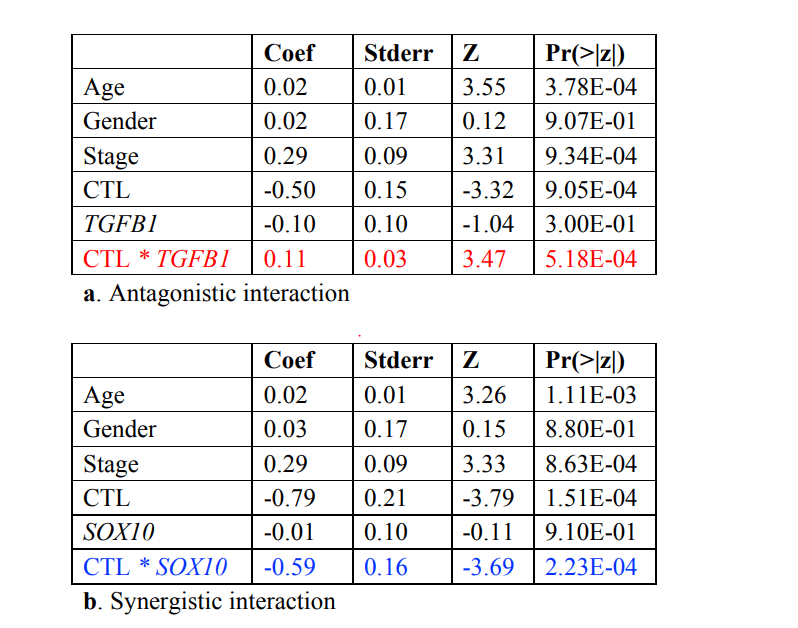# Calculation of dysfunction score in TIDE

#### Introduction

The TIDE score calculated by the TIDE software consists of two parts: dysfunction score and exclusion score. The calculation principle of dysfunction score is that genes with immune disorders have a higher weight, and the dysfunction score can be obtained by multiplying by the amount of expression. The exclusion score is obtained by multiplying the expression amount by the higher weight of immune rejection genes. The weight of immune disorder is obtained by using cancer survival data, and the weight of immune rejection is obtained by using the gene expression of immune rejection samples. This article mainly talks about the calculation of imbalance score.

##### Code explanation
###### Read in the data, prepare the matrix
``````#!/usr/bin/env Rscript
suppressPackageStartupMessages(library(survival))

CTL_genes = c('CD8A', 'CD8B', 'GZMA', 'GZMB', 'PRF1')  # Genes associated with cytotoxic T lymphocytes

writemat = function(result, output)
{
result = result[!is.na(result[,"p"]),,drop=F]
result = cbind(result, FDR)
write.table(result, file=output, sep='\t', quote=F)
}

commands = commandArgs(trailingOnly=T)

# three inputs here
mat = t(readmat(commands)) # expression, mutation, or RPPA matrix
pivot = t(readmat(commands))  # CD8A, CD8B, GZMA, GZMB, PRF1 expression, As a fulcrum
survival = readmat(commands) # clinical information``````
###### Filter data
``````# remove negative survival values
survival = survival[survival[,1] > 0,]  #Remove the error message with negative time to live

output = commands

# Take samples that are present in all data
common = Reduce(intersect, list(rownames(mat),rownames(survival),rownames(pivot)))
print(paste(length(common), "samples"))

# stop at low sample numbers
if(length(common) < 20) stop("two few samples")  # If the sample is too small, it cannot be analyzed

not_included = setdiff(CTL_genes, colnames(pivot))  # The CTL gene and the pivot gene cannot overlap

if(length(not_included) > 0) stop(paste(c("pivot genes", not_included, "are not included"), ' '))

pivot = rowMeans(pivot[common,CTL_genes])  # pivot (cytotoxic T cell expression) data
mat = mat[common,,drop=F]     # Matrix of expressions of all available samples
survival = survival[common,,drop=F]   # Survival information for all available samples

# stop at low death rate
death_rate = sum(survival[,2])/dim(survival)
if(death_rate < 0.1) stop(paste("death rate", death_rate, "is too low"))  # If the mortality rate is too low, the dysfunction obtained is unreliable

# split up survival and background
surv = Surv(survival[,1], survival[,2])  # State of life and time

if(dim(survival) > 2){    #Various variables other than survival status and time
B = survival[,3:dim(survival), drop=F]
}else{
B = survival[,c(), drop=F]
}

# build up regression data space  # Build data
B = cbind(B, pivot, pivot, pivot)
B = as.data.frame(B)
N_B = dim(B)  # Number of columns of data``````
###### Perform survival analysis
``````# test pivot effect first   # Get the regression coefficient of TIL
coxph.pivot = summary(coxph(surv~., data=B[,c(-N_B, -(N_B-1)), drop=F]))\$coef
write.table(coxph.pivot, file=paste0(output, ".pivot"), sep='\t', quote=F)

colnames(B)[N_B-1] = "partner"
colnames(B)[N_B] = "Interaction"

# iterate over features # Get all the characteristic variables and prepare to loop
features = colnames(mat)
N = length(features)

# Matrix of results
result_interaction = matrix(nrow=N, ncol=2, dimnames=list(features, c('z','p')))
result_main = matrix(nrow=N, ncol=2, dimnames=list(features, c('z','p')))
result_partial = matrix(nrow=N, ncol=2, dimnames=list(features, c('z','p')))
result_base = matrix(nrow=N, ncol=2, dimnames=list(features, c('z','p')))

# Loop
for (i in 1:N)
{
title = features[i]
partner = mat[,i]

B[,N_B-1] = partner   # partner就是当前循环的基因的表达量
B[,N_B] = partner * pivot   # partner和pivot的乘积就是interaction

# region 1: model with interaction  # 有interaction的模型，得到的interaction部分的z值就是dysfunction的权重大小
errflag = F
coxph.fit = tryCatch(coxph(surv~., data=B),
error = function(e) errflag <<- T,
warning = function(w) errflag <<- T)

if(!errflag)
{
reg.summary = summary(coxph.fit)\$coef
result_interaction[i,] = reg.summary["Interaction", c("z", "Pr(>|z|)")]
result_main[i,] = reg.summary["partner", c("z", "Pr(>|z|)")]
}

# region 2: model without interaction  # Model without interaction
errflag = F
coxph.fit = tryCatch(coxph(surv~., data=B[,-N_B]),
error = function(e) errflag <<- T,
warning = function(w) errflag <<- T)

if(!errflag)
{
reg.summary = summary(coxph.fit)\$coef
result_partial[i,] = reg.summary["partner", c("z", "Pr(>|z|)")]
}

# region 3: model with only base effect  # Model with only other clinical information
errflag = F
coxph.fit = tryCatch(coxph(surv~., data=B[,c(-N_B, -(N_B-2)), drop=F]),
error = function(e) errflag <<- T,
warning = function(w) errflag <<- T)

if(!errflag)
{
reg.summary = summary(coxph.fit)\$coef
result_base[i,] = reg.summary["partner", c("z", "Pr(>|z|)")]
}
}

writemat(result_interaction, paste0(output, ".interaction"))
writemat(result_main, paste0(output, ".main"))
writemat(result_partial, paste0(output, ".partial"))
writemat(result_base, paste0(output, ".base"))``````
##### Result explanationAs shown in the figure above, the regression coefficient of TGFB1 multiplied by CTL is positive, then it is an antagnonistic gene with CTL, the regression coefficient of SOX10 multiplied by CTL is negative, then it is a positive CTL plays a synergistic role. The zscore of the gene obtained here is the weight of the dysfunction. Multiplying the weight by the expression of the gene and summing it up is the dysfunction of this sample. score

#### Reference

Peng J , Shengqing G , Deng P , et al. Signatures of T cell dysfunction and exclusion predict cancer immunotherapy response[J]. Nature Medicine, 2018.